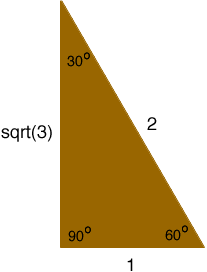Quandaries and Queries Hi, My name is Gary and I have a general math question. I have the length of only 1 side of triangle with angles of 30-60-90 degrees. How can I find the length of the other 2 sides? Thanks for your help. Gary Hi Gary, In most cases if you know you have a right triangle (one of the angles measures 90 degrees) and you know one of the other angles and one of the sides you can use trigonometry to find the lengths of the other two sides. However a triangle with angles 30, 60 and 90 degrees has a property that allows you to solve your question without resorting to trigonometry. The property is that the lengths of the sides of a 30-60-90 triangle are in the ratio 1:2:√3.Thus if you know that the side opposite the 60 degree angle measures 5 inches then then this is √3 times as long as the side opposite the 30 degree so the side opposite the 30 degree angle is 5/√3 inches long. The side opposite the 90 degree angle is twice this long, that is 10/√3 inches long. Penny